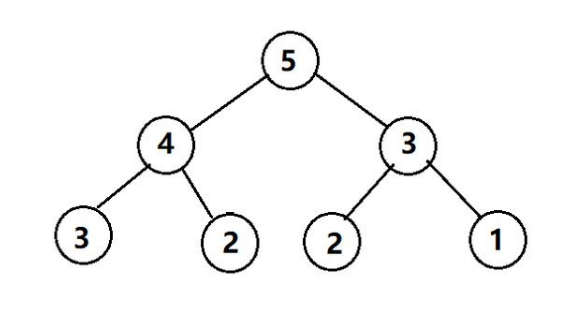5G时代下一个聚合的编程学习网

# Dynamic Programming 基本介绍

Dynamic Programming是五大常用算法策略之一，简称DP，译作中文是“动态规划”，可就是这个听起来高大上的翻译坑苦了无数人，因为看完这个算法你可能会觉得和动态规划根本没太大关系，它对“动态”和“规划”都没有太深的体现。

# Fibonacci 斐波拉契数列动态规划实现``````    public static int simFibonacci(int n) {
if (n == 1) return 1;
else if (n == 2) return 1;
else return simFibonacci(n - 1) + simFibonacci(n - 2);
}``````

## DP策略解决这个问题

``````   //使用数组记录前100个斐波那契数
static int[] arr = new int;

public static int fibonacci(int n) {
if (n == 1) return 1;
else if (n == 2) return 1;
else {
if (n <= 100 && arr[n] != 0) {
return arr[n];
} else {
arr[n] = simFibonacci(n - 1) + simFibonacci(n - 2);
return arr[n];
}
}
}``````

arr数组初始化为0，arr[i]就表示f(i),每次先判断arr[i]是否为0，如果为0则表示未计算过，则递归计算，如果不为0，则表示已经计算过，那就直接返回。

1、确定状态

——-研究最优策略的最后一步
——-化为子问题
2、转移方程

3、初始条件和边界情况

4、计算顺序